# High School Math : Basic Single-Variable Algebra

## Example Questions

1 2 3 4 6 Next →

### Example Question #4 : Solving Inequalities

Solve the inequality for x: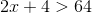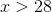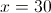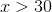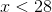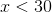Explanation:Subtract 4 from both sides: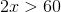Divide both sides by 2:### Example Question #5 : Solving Inequalities

Solve for.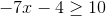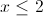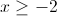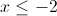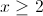Explanation: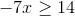Divide both sides by –7. When dividing by a negative value, we must also change the direction of the inequality sign.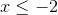### Example Question #6 : Solving Inequalities

Solve for: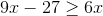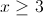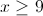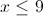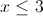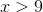Explanation:Move like terms to the same sides: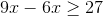Combine like terms: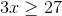Divide both sides by 3: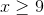### Example Question #52 : Inequalities

Solve for: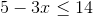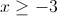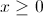Explanation:

Inequalities can be treated like any other equation except when multiplying and dividing by negative numbers. When multiplying or dividing by negative numbers, we just flip the sign of the inequality so that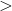becomes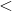, and vice versa.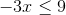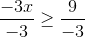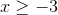### Example Question #1 : Understanding Direct Proportionality

Sarah notices her map has a scale of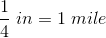.  She measures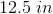between Beaver Falls and Chipmonk Cove.  How far apart are the cities?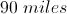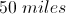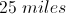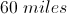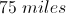Explanation:is the same as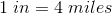So to find out the distance between the cities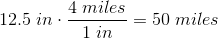### Example Question #2 : Understanding Direct Proportionality

Sunshine paint is made by mixing three parts yellow paint and one part red paint. How many gallons of yellow paint should be mixed with two quarts of red paint?

(1 gallon = 4 quarts)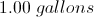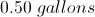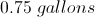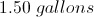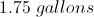Explanation:

First set up the proportion: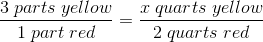x =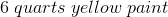Then convert this to gallons: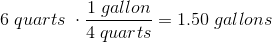### Example Question #3 : Understanding Direct Proportionality

Ifis directly proportional to, andwhen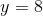, find a formula for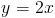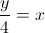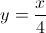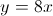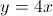Explanation: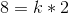sinceandSolving for, you get that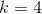Replacingin the original equation, you get the answer of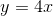### Example Question #51 : Basic Single Variable Algebra

Ifis inversely proportional to, and ifwhen, find a formula for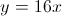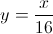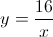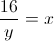Since y is inversely porportional to x, you can use the standard equations of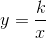where k is a constant. Plugging the given x and y into this equation to solve for k,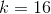. To get the answer, substitute k into the standard equation.